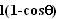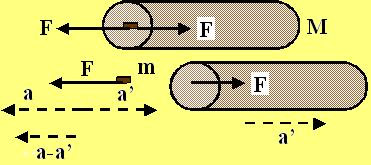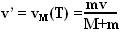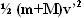## Monday, May 19, 2008

### Irodov Problem 1.162Initially suppose that the bullet comes along with a velocity v. As soon as the bullet hits the cylinder, it gets attached to the cylinder and now the entire (cylinder+bullet) system starts to move towards the right with a velocity v'. Since there are no forces acting on the (bullet+cylinder) system in the horizontal direction, the momentum of the (bullet+cylinder) system must be conserved before and after the collision. In other words,At the end of this solution I will explain the inner workings of the law of conservation of momentum for the more inquisitive students using purely newton's laws.

After the collision the (cylinder+bullet) system starts to swing upwards as shown in the figure. During this process the kinetic energy of the (cylinder+bullet) system is converted into gravitational potential energy. Finally when the system reaches its maximum height, all its kintetic energy is converted into potential energy. Since the strings deflect by and angle, the entire system raises by a height ofand thus gains a potential energy of. The initial kinetic energy of the system right after the bullet fused into the cylinder is given by. So from conservation of energy we have,Inner workings of conservation of momentum
: This part is to show the inner machinery behind the law of conservation of momentum using purely Newton's laws. Suppose that thebullet hits the cylinder with a velocity v. As the bullet enters the cylinder, the material inside the cylinder offer resistance and slows it down to a complete stop. Suppose that the resistance offered by the cylinder is F(t), some arbitrary function of time since it first enters the cylinder.
The bullet offers an equal and opposite reaction force on to the cylinder. Let the deceleration of the bullet in the reference frame of the cylinder be a(t) and let the acceleration of the cylinder be a'(t) relative to an stationary frame of reference. The acceleration of the bullet in the stationary frame of reference will then be a(t)-a'(t).

The equation for the dynamics of the bullet will be,The equation for the dynamics of the cylinder will be,Hence we have,Integrating both sides we have,Suppose that the bullet moved for a very small time T before it came a complete stop i.e. vm(T) =0. So we haveBut this is same as what we got using conservation of momentum in (1)!!! This indicates how beautifully the knowledge of conservation of momentum helps bypass all the tedious inner details of a system to arrive at the same result.

b) The total energy of the (cylinder+bullet) system initially before the collision of the bullet and cylinder is simply the kinetic energy of the bullet given by. After the collision the kinetic energy is given by. The difference is the loss of energy as heat generated by the material resistance that slowed the bullet. So the fraction of energy lost as heat is given by,